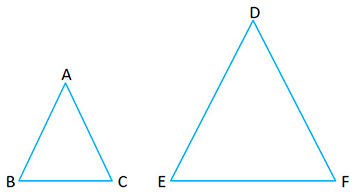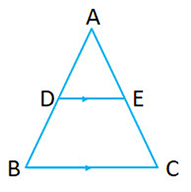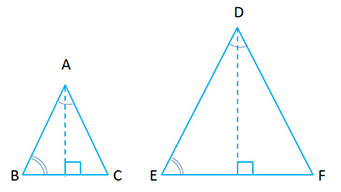Properties of Similar Triangles:If$\triangle ABC \sim \triangle DEF$, then the following holds true:

Corresponding angles are congruent (same measure)$\angle A = \angle D, \ \ \angle B = \angle E, \ \ \angle C = \angle F$

Corresponding sides are all in the same proportion$\frac{AB}{DE}=\frac{BC}{EF}=\frac{AC}{DF}$

Axioms of Similarity of Triangles:

1. SAS (Side Angle Side) Postulate: If two triangles have a pair of corresponding angles equal and the sides including them are proportional, then the two triangles are similar. Or we can also say “Triangles are similar if two sides in one triangle are in the same proportion to the corresponding sides in the other, and the included angles are equal.”

If in$\triangle ABC \ and \ \triangle DEF$$\angle B = \angle E$  and$\frac{AB}{DE}=\frac{BC}{EF}$

Then$\triangle ABC \sim \triangle DEF$

2. AAA (Angle Angle Angle) Postulate. It is also called AA postulate. If two angles are equal, then the third one is equal too. In our discussion, we will use AAA as our nomenclature so that there is no confusion. Or we can say “Triangles are similar if the measure of all three interior angles in one triangle are the same as the corresponding angles in the other.”

If in$\triangle ABC \ and \ \triangle DEF$$\angle A = \angle D$  and$\angle B = \angle E$ (also implies$\angle C = \angle F$

Then$\triangle ABC \sim \triangle DEF$

3. SSS (Side Side Side) Postulate: If in two triangles, the corresponding sides are proportional, then the two triangles are similar.

If in$\triangle ABC \ and \ \triangle DEF$$\frac{AB}{DE}=\frac{BC}{EF}=\frac{AC}{DF}$

Then$\triangle ABC \sim \triangle DEF$

Theorem of Proportionality:

1. If a line is drawn parallel to any side of the triangle, then the line divides the other two sides of the triangle proportionally.Therefore, if$DE \parallel BC \Rightarrow \frac{AD}{BD}=\frac{AE}{CE}$

Conversely, If a line divides the two sides of a triangle  proportionally, the line is parallel to the third side.

Therefore, if$\frac{AD}{BD} = \frac{AE}{CE} \Rightarrow DE \parallel BC$

2. Also because the two triangles are similar i.e.$\triangle ABC \sim \triangle ADE$$\frac{AD}{AB}=\frac{AE}{AC}=\frac{DE}{BC}$

or$\frac{AB}{AD}=\frac{AC}{AE}=\frac{BC}{DE}$

Relation between the areas of two similar triangles:If$\triangle ABC \sim \triangle DEF$ then$\frac{Area \ of \ \triangle ABC}{Area \ of \ \triangle DEF} = \frac{AB^2}{DE^2}=\frac{BC^2}{EF^2}=\frac{AC^2}{DF^2}$

Note:

• A median divides the triangle into two triangles of the same area.

i.e. Area of$\triangle ABD = Area of \triangle ADC = \frac{1}{2} \times Area of \triangle ABC$• If multiple triangles have a common vertex (as shown in the diagram) and their bases are along a straight line, then the following holds true:$\frac{Area \ of \ \triangle ABE}{Area \ of \ \triangle ADC} = \frac{BE}{DC}$$\frac{Area \ of \ \triangle ABD}{Area \ of \ \triangle ABC} = \frac{BD}{BC}$

Maps and Models:

The concept of the similarity applies to the models that we build. The models are similar to the real object just that they  are small (to be manageable). In case of models:$\frac{Height \ of \ the \ model}{Height \ of \ the \ object} = \frac{Length \ of \ the \ model}{Length \ of \ the \ object} = \frac{Width \ of \ the \ model}{Width \ of \ the \ object}$$= k (scale \ factor)$

For a given scale factor$k$:

i)

• If$k \ge 1\Rightarrow$ the transformation is an enlargement
• If$k \le 1 \Rightarrow$ the transformation is a reduction
• If$k = 1\Rightarrow$ the transformation is an identity transformation

ii)

• Each side of the resulting figure (model) is$k$ times the corresponding side of the given figure (object)
• The area of the resulting figure (model) is$k^2$ times the area of the given figure (object)
• In case of solids, the volume of the resulting figure (model) is$k^3$ times the volume of the given object